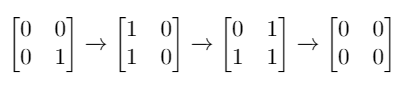## 1284. Minimum Number of Flips to Convert Binary Matrix to Zero Matrix

Given a `m x n` binary matrix `mat`. In one step, you can choose one cell and flip it and all the four neighbours of it if they exist (Flip is changing 1 to 0 and 0 to 1). A pair of cells are called neighboors if they share one edge.

Return the minimum number of steps required to convert `mat` to a zero matrix or -1 if you cannot.

Binary matrix is a matrix with all cells equal to 0 or 1 only.

Zero matrix is a matrix with all cells equal to 0.

Example 1:```Input: mat = [[0,0],[0,1]]
Output: 3
Explanation: One possible solution is to flip (1, 0) then (0, 1) and finally (1, 1) as shown.
```

Example 2:

```Input: mat = []
Output: 0
Explanation: Given matrix is a zero matrix. We don't need to change it.
```

Example 3:

```Input: mat = [[1,1,1],[1,0,1],[0,0,0]]
Output: 6
```

Example 4:

```Input: mat = [[1,0,0],[1,0,0]]
Output: -1
Explanation: Given matrix can't be a zero matrix
```

Constraints:

• `m == mat.length`
• `n == mat.length`
• `1 <= m <= 3`
• `1 <= n <= 3`
• `mat[i][j]` is 0 or 1.

## Rust Solution

``````struct Solution;

impl Solution {
fn min_flips(mut mat: Vec<Vec<i32>>) -> i32 {
let n = mat.len();
let m = mat.len();
let mut res = std::usize::MAX;
Self::dfs(0, 0, &mut mat, &mut res, n, m);
if res == std::usize::MAX {
-1
} else {
res as i32
}
}

fn dfs(start: usize, k: usize, mat: &mut Vec<Vec<i32>>, min: &mut usize, n: usize, m: usize) {
if start == n * m {
if Self::ones(mat, n, m) == 0 {
*min = (*min).min(k);
}
} else {
let r = start / m;
let c = start % m;
Self::flip(r, c, mat, n, m);
Self::dfs(start + 1, k + 1, mat, min, n, m);
Self::flip(r, c, mat, n, m);
Self::dfs(start + 1, k, mat, min, n, m);
}
}

fn flip(i: usize, j: usize, mat: &mut Vec<Vec<i32>>, n: usize, m: usize) {
mat[i][j] = 1 - mat[i][j];
if i > 0 {
mat[i - 1][j] = 1 - mat[i - 1][j];
}
if j > 0 {
mat[i][j - 1] = 1 - mat[i][j - 1];
}
if i + 1 < n {
mat[i + 1][j] = 1 - mat[i + 1][j];
}
if j + 1 < m {
mat[i][j + 1] = 1 - mat[i][j + 1];
}
}

fn ones(mat: &[Vec<i32>], n: usize, m: usize) -> usize {
let mut res = 0;
for i in 0..n {
for j in 0..m {
if mat[i][j] == 1 {
res += 1;
}
}
}
res
}
}

#[test]
fn test() {
let mat = vec_vec_i32![[0, 0], [0, 1]];
let res = 3;
assert_eq!(Solution::min_flips(mat), res);
let mat = vec_vec_i32![];
let res = 0;
assert_eq!(Solution::min_flips(mat), res);
let mat = vec_vec_i32![[1, 1, 1], [1, 0, 1], [0, 0, 0]];
let res = 6;
assert_eq!(Solution::min_flips(mat), res);
let mat = vec_vec_i32![[1, 0, 0], [1, 0, 0]];
let res = -1;
assert_eq!(Solution::min_flips(mat), res);
}
``````

Having problems with this solution? Click here to submit an issue on github.